# Open Circuit: What is it? (And How Does it Differ To a Short Circuit)

Contents

## What is an Open Circuit?

An open circuit is defined as an electric circuit in which current does not flow. Current can only flow in a circuit if it finds a continuous path—known as a “closed circuit”. If there’s a break anywhere in the circuit, you have an open circuit, and current can not flow.

In an open circuit, the two terminals are disconnected. Hence the continuity of the circuit is broken. But while current cannot flow through the circuit, there is some voltage drop between two points of a circuit.

Hence in an open circuit, the current flowing through the circuit is zero, and voltage is present (non-zero).

Now Power is equal to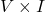, and the current is equal to zero.

Hence power is also equal to zero, and no power dissipates from an open circuit.

The resistance of an open circuit is discussed in more detail below.

## Open Circuit Resistance

The behavior of a resistor is given by Ohm’s law. The voltage across the resistor is proportional to the current. Hence, the equation of ohm’s law is,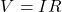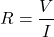In an open circuit condition, the current is zero (I = 0).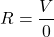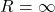Hence, for any value of voltage, the resistance is infinite in open circuit conditions.

## Open Circuit vs Short Circuit

In the basics of electrical engineering, open circuit and short circuit are two special configurations with opposite behavior.

Both concepts represent the connection of two terminals of the circuit. So, the question is what is the difference between an open circuit and a short circuit?

In open-circuit conditions, the current passing through the circuit is zero. While in the case of short circuit conditions, a very high amount (infinite) of current passing through the circuit.

The resistance between two terminals of open circuits is infinite. And the resistance between two terminals of shorts circuits is ideally zero. But practically there is very low resistance.

The voltage across terminals of the open circuit is equal to supply voltage. And in the short circuit, the voltage across short circuit terminals is zero.

When a circuit is running in normal condition and the current passing through the components, this condition is known as a close circuit. The current only flows when a close path is created. In a close path, a current will flow from the negative to the positive polarity of the voltage.

The difference between a close circuit, open circuit, and short circuit is as below figure.

## Open Circuit Example

In most cases, open circuit causes due to breaking in a conductor. If the circuit is not closed and there is a break in anywhere in the loop, the current cannot flow. It creates an open circuit condition.

For a simple example, let’s a bulb charged with a battery through a switch. The circuit diagram is shown below figure.

When a switch is open, it will break a path. It means the current cannot flow in this condition. And this is an open circuit condition.

But when you close a switch, it will make a close path. And some amount of current will flow through the load (bulb). This is a normal condition or closed circuit.

Due to any conditions, if positive and negative terminals of a battery are connected, the current has a close path to flow. But there is no load (resistance). Hence, it causes a short circuit and a high amount of current flow.

Want To Learn Faster? 🎓
Get electrical articles delivered to your inbox every week.
No credit card required—it’s 100% free.